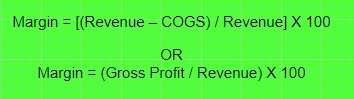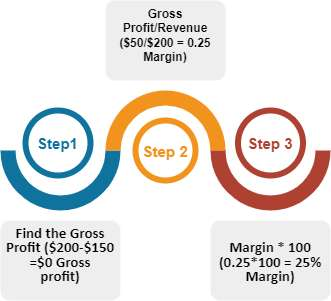"Profit margin" is an important term and concept in the world of commerce and economic growth. What is a profit margin? How to calculate profit margin? This article will describe the idea, its definition, and the numerous profit margin formulas you may apply. The examples given in this post will make it abundantly evident how simple it is to understand profit margin calculation. The definition is basic; the margin formula is simple as well.

A profit margin tells you all you need to know about how flexible a business can be. It is one of the most common metrics used by investors to judge the profitability of a proposed or current investment.

It's amazing how a simple statement with a simple profit margin formula or formulas: one for the gross profit margin, one for the net profit margin, one for the operating profit margin, and so on can be so widely used that it would be easy to understand what profit margin is all about.

1. Profit margin reveals the amount of money your firm makes, its general health, and whether the business is having issues.1. The higher the profit margin, the better, but a high gross margin and a low net margin signify that something has to be looked into further.
2. Business owners should always know how their company spends money to maximize revenues.
3. Profit margins differ by industry and should only be evaluated with businesses in the same industry.
4. If your calculations indicate issues, you should be mindful of idling expenditures. Reducing these expenses is a good cash flow strategy.

## What is Profit Margin?

Profit Margin determines the profitability of any business or company. It is measured as a percentage and indicates how much of each dollar in sales or services your business retains as profit. When net income is split by net sales or revenue, the profit margin is what remains—subtracting the company's costs from its total revenue yields net income, also known as net profit.

You may examine your profit margin in a few different ways:

• Net profit margin
• Gross Profit Margin
• Operating Profit Margin### Understanding Types of Profit Margin with Formulas and Examples

Gross profit margins, operating profit margins, and net profit margins are the three different types of profit margins. Each one gives you a glimpse into how well a business is running.

#### Operating Profit Margin

COGS, or cost of goods sold, refers to costs associated with creating or manufacturing goods intended for sale, including salaries, supplies, and other directly attributable costs. After deducting the cost of products sold, profit is defined as all money left over (COGS). COGS does not include debt, taxes, operational or administrative costs, or one-time purchases like equipment.

The ratio of gross profit to total revenue, or gross profit margin, indicates the portion of each dollar of sales that is kept as profit after deducting the cost of production.

The amount of profit an organization makes as a percentage of its total revenue is determined by its net profit margin. A company's profit per dollar is expressed as a percentage by dividing net income by revenue.

Net income, which includes the cost of goods sold (COGS), operating expenditures, debt service, taxes, one-time payments, investments, and secondary activities, is the total revenue left over after deducting all costs and income sources.

Operating profit margin, also known as EBIT margin, operating income margin, and return on sales, is the percentage of gross profit after all overhead, operating, administrative, and sales costs are deducted.

Debts, taxes, and other non-operational expenditures are not included in the computation. In other words, operational profit margin determines the proportion of each dollar left over after paying for all necessary expenses by considering all costs associated with operating a firm daily.

Formula

Gross profit margin formula: Gross profit margin = ((Net sales – COGS) / Net sales) * 100

Net Profit Margin Formula: Net profit margin = (Net income / Revenue) * 100

Operating Profit Margin Formula: Operating profit margin = (Operating income / Revenue) * 100

Example

A company's gross profit margin will be 60% if its revenue is \$10,000 and its cost of goods sold is \$4,000.

A company's net profit margin will be 33.33% if it has a net income of \$5,000 and revenue of \$15,000.

An organization's operating profit margin is 10% if it earns \$10,000 in operating income on \$100,000 in revenue.### What is the Profit Margin Formula?

You should use the net profit margin formula to calculate your company's total profit margin. To get the overall profit margin for your company, use the following formula:#### How To Calculate Profit Margin: Step-By-Step Guide

1. Firstly, figure out your cost of goods sold (COGS) and the income you'll get from selling these products.
2. Then, subtract the cost from the income to determine the gross profit.
3. Next, divide revenue by gross profit.
4. Finally, put it in percentage form.

This is how profit margin calculations work.

Are you ready to see how profit margin is calculated in action? Let's see how to calculate profit margin using the following example:

Assume Company C generates \$100,000 in sales and spends \$50,000 overall over a year.

When we enter these numbers into the profit margin formula, we receive a net income of \$50,000 divided by net sales of \$100,000.

Therefore, Company C has a profit margin of 50%, or in other words, it makes 50 cents for every dollar of sales.

### What Is A Good Profit Margin?

You might be wondering, "What is a good profit margin?" Generally, a 10% net profit margin is regarded as typical, a 20% margin as high (or "excellent"), and a 5% margin as poor. However, a decent margin will differ significantly per industry.

##### Note: These criteria differ significantly by sector and firm size, and several other factors may also have an influence.Now that we are aware of what a healthy profit margin is, let's examine the highest profit margins per industry as of 2022:

 INDUSTRY PROFIT MARGINS Finance 32% Software (entertainment) 29.04% Information Services 16.92% Transportation 28.90% Computers and Peripherals 18.72% Tobacco 20.58% Software (System and Application) 19.66%

### Why is Profit Margin Important?

Profit margins enable financial analysts to evaluate a firm's strength and industry competitiveness. Decision-makers can use it to decide whether to concentrate on boosting sales volume, profit margins, or both at once.

Sometimes raising one may lower the other. When organizations start to scale their operations, it's not unusual for them to produce a smaller profit margin.### What is Margin?

The revenue you generate after paying COGS is shown by a margin, often known as gross profit margin. Your margin is the discrepancy between your profit and your profit cost.

Margin FormulaThe margin formula calculates how much of each dollar in revenue is left over after expenditures are covered. The percentage of income you keep from a sale increases with margin size.

#### How to Calculate Margin

If you are looking for a step-by-step process to how to calculate margin, then you can go through the steps below:

1. Firstly, start by calculating your gross profit, which is the difference between sales and COGS.
2. Then, calculate the gross profit as a percentage of revenue.
3. Divide your gross profit by your revenue to determine this.
4. Finally, multiply the sum by 100 to get your margin percentage#### Gross Profit Margin vs Operating Profit Margin

If you wish to analyze a company's financial performance on the income statement or P&L, you must comprehend two fundamental financial terms: gross margin and operating margin (profit and loss statement).

The gross profit margin of a company is defined as net sales less the total cost of goods sold. This measure indicates how much profit a corporation produces before deducting general and administrative expenses. The operating profit margin, refers to any profit made after paying for variable costs such as wages and raw materials.#### The Bottom Line:

Profit margins are used to evaluate how effectively a company's management generates profits. It's useful to compare profit margins over time and with companies in the same industry.

Net profit margin is an extremely valuable indicator to present with potential investors since it gives a glimpse of your company's entire profitability.

#### FAQs

1. Use the decimal form of 20%, which is 0.2.
2. Subtract 0.2 from 1 to obtain 0.8.
3. Multiply the original price of your item by 0.8.
4. The result is the amount you should charge for a 20% profit margin.

A company's profit margin may be calculated by deducting its cost of goods sold (COGS) from its sales and dividing the result by the total revenue. To get a percentage, multiply that value by 100. So, a corporation with \$1,000 in revenue and \$200 in COGS has a profit margin of 80%, or (\$1,000 - \$200) \$1,000.

The net profit margin formula is most likely the most useful profit margin formula because it is calculated using net income. Investors, creditors, and corporate decision-makers care about the company's bottom line.

The concept of a good margin varies greatly depending on the industry and size of the business. Nonetheless, a 20% margin is considered excellent (or "good"), and a 5% margin is considered insufficient.

You may calculate profit on sale using the following formula:

Total Revenue - Total Expenses = Profit.Live Support

+1-860-497-7164